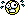A Flash Developer Resource Site

# Thread: Needing Help in retrieving value from array

1. ## Needing Help in retrieving value from array

Hello all, any help will be appreciated
so here i got a array of number
i want to filter the array
for example
the array would be 5, 10, 20, 30 , 40 , 50 , 66 , 77
i would like to filter it it this way
if the array is between number 4 - 11 then count how many is array.. from the example above we can see there are 2 (5,10) after that store the result in textbox then continue with filtering number 12-40 and so on until the end of array..

any idea guyReply With Quote

2. Hi,

PHP Code:
``` var numbers:Array = new Array();var amounts:Array = new Array();numbers = [5, 10, 29, 30, 40, 50, 66, 77];amounts = [[4, 11], [12, 40], [41, 77]];for (var i:Number = 0; i < numbers.length; i++){    var item:Number = numbers[i];    for (var j:Number = 0; j < amounts.length; j++)    {        var low:Number = amounts[j];        var high:Number = amounts[j];        if (item >= low && item < high)        {            trace(item + " is greater than " + low + " and lower than " + high);            trace("----------");        }    }}  ```Reply With Quote

3. HI,

Expanding on that, try this, paste into a new AS2 document and test.
PHP Code:
``` var numbers:Array = new Array();var amounts:Array = new Array();var outcome:Array = new Array();var addOutput:String = "";numbers = [5, 10, 20, 30, 40, 50, 66, 77];amounts = [[4, 11], [12, 40], [41, 77]];outcome = [[], [], []];// same length as amounts length within.for (var i:Number = 0; i < numbers.length; i++){    var item:Number = numbers[i];    for (var j:Number = 0; j < amounts.length; j++)    {        var low:Number = amounts[j];        var high:Number = amounts[j];        if (item >= low && item < high)        {            outcome[j].push(item);            //trace(item + " is greater than " + low + " and lower than " + high);            //trace("----------");        }    }}for (var q:Number = 0; q < outcome.length; q++){    for (var d:Number = 0; d < outcome[q].length; d++)    {        if (d < outcome[q].length - 1)        {            addOutput += outcome[q][d] + ", ";        }        else        {            addOutput += outcome[q][d];        }    }    trace(outcome[q].length + " are within " + amounts[q] + " - " + amounts[q] + " these are " + addOutput);    addOutput = "";}  ```Reply With Quote

4. Hi again,

Been busy wasting time, expanding on that again.

PHP Code:
``` var numbers:Array = new Array();var amounts:Array = new Array();var outcome:Array = new Array();var i:Number = 0;var j:Number = 0;numbers = [4, 10, 12, 13, 14, 18, 19, 22, 24, 30, 47, 50, 66, 94, 99];amounts = [[4, 11], [12, 40], [41, 80], [81, 96], [97, 150]];/*** fill result array with empty arrays ***/for (i = 0; i < amounts.length; i++){    var populateOutcome:Array = new Array();    outcome.push(populateOutcome);}trace(outcome.length + " arrays created inside of outcome array.");trace("------------------------------------------");for (i = 0; i < numbers.length; i++){    var item:Number = numbers[i];    for (j = 0; j < amounts.length; j++)    {        var low:Number = amounts[j];        var high:Number = amounts[j];        if (item >= low && item <= high)        {            outcome[j].push(item);            //trace(item + " >= " + low + " && <= " + high);        }    }}for (i = 0; i < outcome.length; i++){    var doGrammar1:String = "";    var doGrammar2:String = "";    if (outcome[i].length < 2)    {        doGrammar1 = " number is within number range ";        doGrammar2 = " the number is ";    }    else    {        doGrammar1 = " numbers are within number range ";        doGrammar2 = " the numbers are ";    }    trace(outcome[i].length + doGrammar1 + "(" + amounts[i] + " - " + amounts[i] + ")\t" + doGrammar2 + " - [" + outcome[i] + "]");}  ```Reply With Quote

5.Reply With Quote

6. I think that you guys have missed the convenient option of using array.filter(): http://board.flashkit.com/board/show...ilter()-method is an example I found discussing this.Reply With Quote

7. Hi,

Can you make an example of how it will work with this guys problem ( even though he has never come back ) nor explained any further about how he wanted the filter to continue.Reply With Quote

8. I just realized two things. 1) it would be interesting to try and make this kind of thing into a resource to make doing this kind of thing easier. 2) as2 doesn't have a built in filter. So doing it your way is really the only way. That page is still quite decent though.Reply With Quote

actionscript2, array####Posting Permissions

• You may not post new threads
• You may not post replies
• You may not post attachments
• You may not edit your posts
•

 » Home » Movies » Tutorials » Submissions » Board » Links » Reviews » Feedback » Gallery » Fonts » The Lounge » Sound Loops » Sound FX » About FK » Sitemap